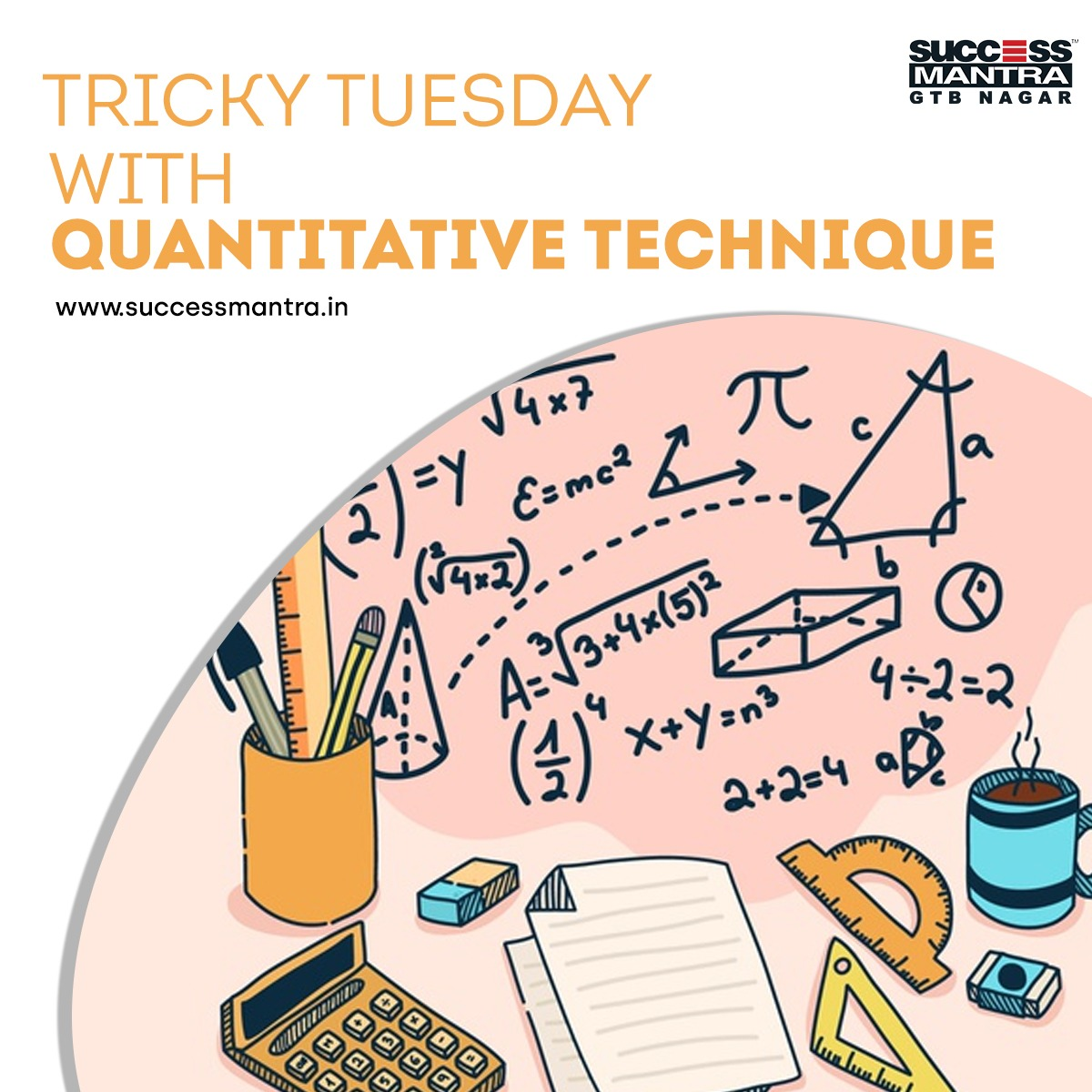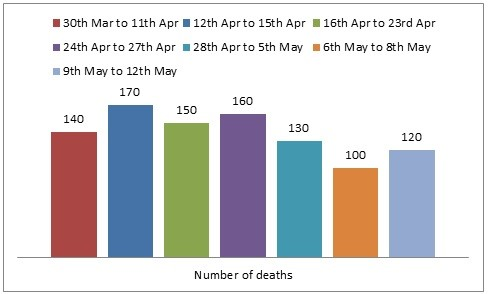## Questions On Quantitative Techniques SMQTQ018Directions for questions 1 and 2 : These questions are based on the graph given below, which shows the number of deaths due to fire accidents in the summer of 1992.1. The average number of deaths per day during the given period is

a. 22.04
b. 22.5
c. 23.09
d. 21.08

2. Out of the total deaths from 30th March to 8th May, if 22% occurred in place X and 10% of them are because of cigarettes, then the number of fire accidents for the given period in place X because of cigarettes is approximately.

a. 19
b. 20
c. 22
d. 23

3. A train can travel 50% faster than a car. Both start from point A at the same time and reach point B 75 kms away from A at the same time. On the way, however, the train lost about 12.5 minutes while stopping at the stations. The speed of the car is:

a. 100kmph
b. 150kmph
c. 120kmph
d. 90kmph

4. An automobile financier claims to be lending money at simple interest, but he includes the interest every six months for calculating the principal. If he is charging an interest of 10%, the effective rate of interest becomes:

a. 8%
b. 10.25%
c. 11%
d. 12%

1. Option – a

Exp: 970/44 = Total deaths/Total days = 22.04.

1. Option – a

Exp: 850 x 0.22 x 0.1 = 19.

1. Option – c

Let speed of the car be x kmph.

 Then, speed of the train = 150 x = 3 x kmph. 100 2

 75 - 75 = 125 x (3/2)x 10 x 60

 75 - 50 = 5 x x 24

 x = 25 x24 = 120 kmph. 5

1. Option – b

Let the sum be Rs. 100. Then,

 S.I. for first 6 months = Rs. 100 x 10 x 1 = Rs. 5 100 x 2

 S.I. for last 6 months = Rs. 105 x 10 x 1 = Rs. 5.25 100 x 2

So, amount at the end of 1 year = Rs. (100 + 5 + 5.25) = Rs. 110.25

Effective rate = (110.25 - 100) = 10.25%